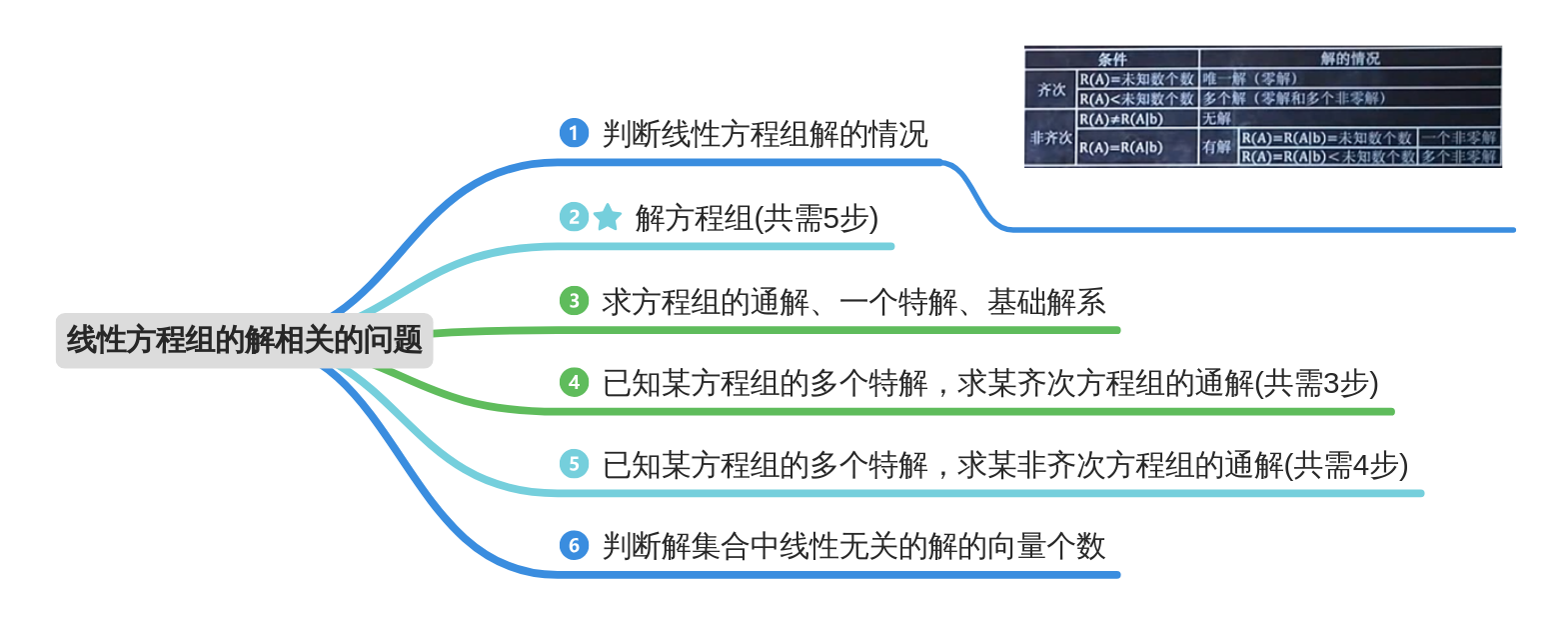# 线性代数复习笔记(二)【矩阵的初等变换】

## 1.矩阵初等变换

#### 1.1初等行变化

1. 对换两行($r_{i} \leftrightarrow r_{j}$);
2. 数k(k$\neq$0)乘以某一行的所有元(第$i$行乘以k，即$r_{i}*k$);
3. 某行的所有元加上另一行的对应的所有元的k倍。(第$j$行的$k$倍加到第$i$行上，即$r_{i}+k*r_{j}$).

#### 1.2初等列变换

• 将上面的定义的中的”行“换成”列“，就是初等列变换.

#### 1.3初等变换

• 初等行变化与初等列变换，统称为初等变换.
• A $\stackrel{有限次初等行变换}{\longrightarrow}$B，则记为A与B行等价;
• A $\stackrel{有限次初等列变换}{\longrightarrow}$B，则记为A与B列等价;
• A $\stackrel{有限次初等变换}{\longrightarrow}$B，则记为A与B等价，记为A ~ B.

1. 行阶梯型矩阵(不唯一);
2. 行最简形矩阵(唯一);
3. 标准型。特点：左上角是一个单位矩阵，其余的全是0.

1. A(mxn)矩阵，对A施行一次行变换，相当于A的左边乘相应的m阶初等矩阵；对A施行一次列变换，相当于A的右边乘相应的n阶初等矩阵.
2. 方阵A可逆的充分必要条件是：A~B.考试常考题型

$$通过该性质求解A^{-1}：\\ PA=B,PE=P\Leftrightarrow P(A,E)=(B,P)\\$$

$$通过该性质求解AX=B：\\ X=A^{-1}B:PA=E,PB=PB\Leftrightarrow P(A,B)=(E,PB)=(E,A^{-1}B)\\$$

## 2.矩阵的秩

### 2.1秩的概念

• 有一个有5个向量组成的方阵，如果这5个向量中最多有3个向量互不相关，就说这个矩阵的秩为3；如果这5个向量中最多有4个向量互不相关，就说这个矩阵的秩为4；如果这5个向量中5个向量都互不相关，就说这个矩阵满秩。满秩，就是组成矩阵的所有向量都线性无关。
• 先说解决数学本身的一个实用问题。要解一个方阵组成的线性代数方程，如果矩阵满秩，方程才有唯一解。即：线性代数方程组有唯一解的条件是：矩阵满秩。否则，方程就无解。
• 现代控制理论中的一个实用问题。线性系统有一个矩阵，叫能控性矩阵。如果这个矩阵是满秩的，系统的状态就完全能控制；如果不满秩，系统的状态就不完全能控制。

### 2.2矩阵的性质

• $A$的秩$R(A)$就是$A$的非零子式的最高阶数。规定：0矩阵的秩为0.
• n阶矩阵$A$，$|A|\neq$0时$R(A)=n$；$|A|=0$时，$R(A)<n$.（可逆矩阵的秩等于矩阵的阶数，不可逆矩阵的秩小于矩阵的阶数。可逆矩阵称为满秩矩阵，不可逆矩阵称为降秩矩阵

1. $0\leq R(A_{m*n})\leq min\{m,n \}$.
2. $R(A^T)=R(A)$.
3. 若$A$~$B$,则$R(A)=R(B)$.
4. 若$P$、$Q$可逆，则$R(PAQ)=R(A)$.
5. $max\{ R(A),R(B)\} \leq R(A,B)\leq R(A)+R(B)$.特殊情况：当$B=b$为非零列向量时，$R(A)\leq R(A,b)\leq R(A)+1$.
6. $R(A+B)\leq R(A)+R(B)$.
7. $R(AB)\leq min\{R(A),R(B)\}$.
8. 若$A_{m*n}B_{n*l}=0$，则$R(A)+R(B)\leq n$.

## 3.线性方程组的解### 3.2解方程组(5步)

1. 若为齐次方程组，求$R(A)$;若为非齐次方程组，求$R(A)与R(A|b)$,判断是否有解；
2. 将增广矩阵变换为行最简型矩阵;
3. 将②步的矩阵变回方程组;
4. 设n个未知数$k_1,k_2,\cdots ,k_n$(n为未知数个数$-$R)
5. 将方程组整理为标准形式，再用$k_1,k_2,\cdots ,k_n$依次替代倒数第一，第二，$\cdots$，第n个未知数。

$$\begin{cases} x_1+2x_2+x_3-x_4=0,\\ 3x_1+6x_2-x_3-3_4=0,\\ 5x_1+10x_2+x_3-5x_4=0. \end{cases}$$

①:求解增广矩阵的秩$R(A|b)$;

$$R(A|B)= \left\{ \begin{matrix} 1 & 2 & 1 & -1 & 0\\ 3 & 6 & -1 & -3 & 0\\ 5 & 10 & -1 & -5 & 0\\ \end{matrix} \right\} \stackrel{r_2-3r_1\\r3-5r_1\\r3-r_2\\r2/(-4)}\longrightarrow \left\{ \begin{matrix} 1 & 2 & 0 & -1 & 0\\ 0 & 0 & 1 & 0 & 0\\ 0 & 0 & 0 & 0 & 0\\ \end{matrix} \right\}$$

②:变换为行最简型矩阵如上;
③:将矩阵代回方程组;

$$\begin{cases} x_1+2x_2+-x_4=0\\ x_3=0 \end{cases}$$

④:设未知数。n = 4-R=2.设$x_4=k_1,x_2=k_2$;
⑤:将方程组转换为标准形式。

$$\begin{cases} x_1+2x_2+-x_4=0\\ x_3=0 \end{cases} \longrightarrow \begin{cases} x_1=-2k_2+k_1\\ x_2=k_2\\ x_3=0\\ x_4=k_1 \end{cases}$$

$$\left\{ \begin{matrix} x_1\\ x_2\\ x_3\\ x_4 \end{matrix} \right\} =k_1 \left\{ \begin{matrix} 1\\ 0\\ 0\\ 1 \end{matrix} \right\} + k_2\left\{ \begin{matrix} -2\\ 1\\ 0\\ 0 \end{matrix} \right\} (c_1,c_2\in R)$$

### 3.3求方程组的通解、一个特解、基础解系

• 通解即3.2所求；
• 特解则令k为实际的数即可；
• 基础解系

$$\alpha_1 = k_1 \left\{ \begin{matrix} 1\\ 0\\ 0\\ 1 \end{matrix} \right\} \quad \alpha_2=k_2\left\{ \begin{matrix} -2\\ 1\\ 0\\ 0 \end{matrix} \right\}$$

$\alpha_1,\alpha_2$即为一组基础解系。

### 3.6判断解集合中线性无关的解的向量个数

• 齐次方程：未知数个数(矩阵列数)-秩
• 非齐次方程：未知数个数-秩+1
Last modification：May 28th, 2019 at 06:07 pm